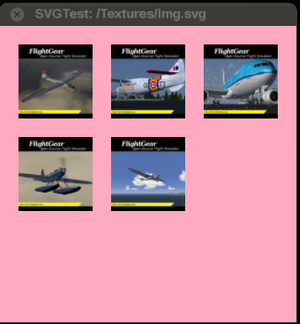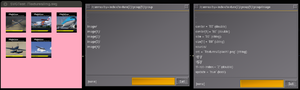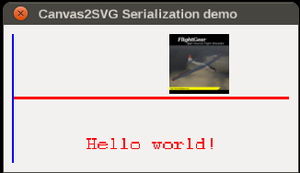# Howto:Serializing a Canvas to SVG

## Background

This was inspired by recurring discussions on the forum to easily reuse existing Canvas based MFDs and show them in a browser or render them remotely using a Python script. The easiest way to do so would be treating a Canvas as a SVG file, simply because most Canvas based MFDs already use SVG files internally. These SVG files are obviously static and parsed by the Nasal svg.nas module to turn them into Canvas properties. However, the opposite would also be possible, all that would require is traversing a canvas node in the tree and iterating over all its child nodes, parsing its properties/attributes to come u p with a corresponding SVG equivalent.

For starters, it makes sense to merely focus on MFDs that are already using SVG files, because instead of actually traversing the full canvas, we can simply let the http server serve the original SVG file itself, which greatly simplifies the whole thing.

However, the next step would actually be traversing an existing Canvas node in the property tree, and then iterating over its child nodes to map certain properties back to their SVG equivalent.

This should work reasonably well, because the module converting svg markup into canvas properties also is implemented in Nasal, and can be easily extended.

For starters we can prototype all of this in scripting space - at some point however, it would make more sense to move this back into C++ by introducing a corresponding `virtual void std::stringstream& serialize()` methid at the Canvas::Element level and providing a mechanism to allow a Canvas to be serialized (and streamed) asynchronously.

And in fact, boost has helpers to deal with SVG processing  

## Example

We will be working with an example taken from another tutorial, one showing a few raster images (splash screens) using fairly simple SVG markup:screenshot showing Canvas GUI dialog with SVG image loaded referencing raster images from \$FG_ROOT/Textures
```<svg xmlns="http://www.w3.org/2000/svg" xmlns:xlink="http://www.w3.org/1999/xlink">
<image x="20" y="20" width="80" height="80" xlink:href="Textures/Splash1.png" />
<image x="120" y="20" width="80" height="80" xlink:href="Textures/Splash2.png" />
<image x="220" y="20" width="80" height="80" xlink:href="Textures/Splash3.png" />
<image x="20" y="120" width="80" height="80" xlink:href="Textures/Splash4.png" />
<image x="120" y="120" width="80" height="80" xlink:href="Textures/Splash5.png" />
</svg>
```

This is referencing 5 different splash screen textures found in \$FG_ROOT/Textures.

We will be using the patched SVG parser to create the properties under /canvas/texture[x] for us, which we will then try to serialize back to a matching SVG file.

When the patched svg module parses the SVG file, it comes up with a property hierarchy according to this, using props.dump():

`props.dump(root._node);`Screenshot showing how the patched svg.nas module turns svg/image tags into the corresponding canvas properties, showing two Property browser dialogs illustrating the internal representation, which can be used to help with Howto:Serializing a Canvas to SVG.
```group {NONE} = nil
group/image {NONE} = nil
group/image/source {NONE} = nil
group/image/source/normalized {BOOL} = 1
group/image/source/right {FLOAT} = 1
group/image/source/bottom {FLOAT} = 1
group/image/tf {NONE} = nil
group/image/tf/m {DOUBLE} = 1
group/image/tf/m {DOUBLE} = 0
group/image/tf/m {DOUBLE} = 0
group/image/tf/m {DOUBLE} = 1
group/image/tf/m {DOUBLE} = 20
group/image/tf/m {DOUBLE} = 20
group/image/size {STRING} = 80
group/image/size {STRING} = 80
group/image/src {STRING} = Textures/Splash1.png
group/image/update {BOOL} = 1
group/image/center {DOUBLE} = 60
group/image/center {DOUBLE} = 60
group/image/tf {NONE} = nil
group/image/tf/m {DOUBLE} = 1
group/image/tf/m {DOUBLE} = 0
group/image/tf/m {DOUBLE} = 0
group/image/tf/m {DOUBLE} = 1
group/image/tf/m {DOUBLE} = 0
group/image/tf/m {DOUBLE} = 0
group/image/tf-rot-index {DOUBLE} = 2
group/image {NONE} = nil
group/image/source {NONE} = nil
group/image/source/normalized {BOOL} = 1
group/image/source/right {FLOAT} = 1
group/image/source/bottom {FLOAT} = 1
group/image/tf {NONE} = nil
group/image/tf/m {DOUBLE} = 1
group/image/tf/m {DOUBLE} = 0
group/image/tf/m {DOUBLE} = 0
group/image/tf/m {DOUBLE} = 1
group/image/tf/m {DOUBLE} = 120
group/image/tf/m {DOUBLE} = 20
group/image/size {STRING} = 80
group/image/size {STRING} = 80
group/image/src {STRING} = Textures/Splash2.png
group/image/update {BOOL} = 1
group/image/center {DOUBLE} = 160
group/image/center {DOUBLE} = 60
group/image/tf {NONE} = nil
group/image/tf/m {DOUBLE} = 1
group/image/tf/m {DOUBLE} = 0
group/image/tf/m {DOUBLE} = 0
group/image/tf/m {DOUBLE} = 1
group/image/tf/m {DOUBLE} = 0
group/image/tf/m {DOUBLE} = 0
group/image/tf-rot-index {DOUBLE} = 2
group/image {NONE} = nil
group/image/source {NONE} = nil
group/image/source/normalized {BOOL} = 1
group/image/source/right {FLOAT} = 1
group/image/source/bottom {FLOAT} = 1
group/image/tf {NONE} = nil
group/image/tf/m {DOUBLE} = 1
group/image/tf/m {DOUBLE} = 0
group/image/tf/m {DOUBLE} = 0
group/image/tf/m {DOUBLE} = 1
group/image/tf/m {DOUBLE} = 220
group/image/tf/m {DOUBLE} = 20
group/image/size {STRING} = 80
group/image/size {STRING} = 80
group/image/src {STRING} = Textures/Splash3.png
group/image/update {BOOL} = 1
group/image/center {DOUBLE} = 260
group/image/center {DOUBLE} = 60
group/image/tf {NONE} = nil
group/image/tf/m {DOUBLE} = 1
group/image/tf/m {DOUBLE} = 0
group/image/tf/m {DOUBLE} = 0
group/image/tf/m {DOUBLE} = 1
group/image/tf/m {DOUBLE} = 0
group/image/tf/m {DOUBLE} = 0
group/image/tf-rot-index {DOUBLE} = 2
group/image {NONE} = nil
group/image/source {NONE} = nil
group/image/source/normalized {BOOL} = 1
group/image/source/right {FLOAT} = 1
group/image/source/bottom {FLOAT} = 1
group/image/tf {NONE} = nil
group/image/tf/m {DOUBLE} = 1
group/image/tf/m {DOUBLE} = 0
group/image/tf/m {DOUBLE} = 0
group/image/tf/m {DOUBLE} = 1
group/image/tf/m {DOUBLE} = 20
group/image/tf/m {DOUBLE} = 120
group/image/size {STRING} = 80
group/image/size {STRING} = 80
group/image/src {STRING} = Textures/Splash4.png
group/image/update {BOOL} = 1
group/image/center {DOUBLE} = 60
group/image/center {DOUBLE} = 160
group/image/tf {NONE} = nil
group/image/tf/m {DOUBLE} = 1
group/image/tf/m {DOUBLE} = 0
group/image/tf/m {DOUBLE} = 0
group/image/tf/m {DOUBLE} = 1
group/image/tf/m {DOUBLE} = 0
group/image/tf/m {DOUBLE} = 0
group/image/tf-rot-index {DOUBLE} = 2
group/image {NONE} = nil
group/image/source {NONE} = nil
group/image/source/normalized {BOOL} = 1
group/image/source/right {FLOAT} = 1
group/image/source/bottom {FLOAT} = 1
group/image/tf {NONE} = nil
group/image/tf/m {DOUBLE} = 1
group/image/tf/m {DOUBLE} = 0
group/image/tf/m {DOUBLE} = 0
group/image/tf/m {DOUBLE} = 1
group/image/tf/m {DOUBLE} = 120
group/image/tf/m {DOUBLE} = 120
group/image/size {STRING} = 80
group/image/size {STRING} = 80
group/image/src {STRING} = Textures/Splash5.png
group/image/update {BOOL} = 1
group/image/center {DOUBLE} = 160
group/image/center {DOUBLE} = 160
group/image/tf {NONE} = nil
group/image/tf/m {DOUBLE} = 1
group/image/tf/m {DOUBLE} = 0
group/image/tf/m {DOUBLE} = 0
group/image/tf/m {DOUBLE} = 1
group/image/tf/m {DOUBLE} = 0
group/image/tf/m {DOUBLE} = 0
group/image/tf-rot-index {DOUBLE} = 2
```

The next step is determining how we can traverse each element to arrive back at the original SVG shown above:

```foreach(var img; svg_symbol._node.getChildren("image")) {
var imgHash = img.getValues();
print("Found image, source is:", imgHash.src);
}```

To actually come up with the correct image tags, we now need to obtain the values for the corresponding attributes, e.g.:

• x="120" => /tf/m
• y="120" => /tf/m
• width="80" => size
• height="80" => size

## Test CanvasScreenshot showing a Canvas GUI dialog with an image element showing a splash screen texture, a Canvas text node and two canvas paths - used for testing a simple serializatition scheme to turn a Canvas into SVG markup.

Our test canvas will be fairly simple, it will show a text node, an image node and two Canvas Path elements. For starters, our goal will be to simply convert this to SVG markup so that we can display that in a browser.

```var (width,height) = (320,160);
var title = 'Canvas2SVG Serialization demo';

var window = canvas.Window.new([width,height],"dialog").set('title',title);

var myCanvas = window.createCanvas().set("background", canvas.style.getColor("bg_color"));

var root = myCanvas.createGroup();

# create an image child for the texture
var img = root.createChild("image")
.setFile("Textures/Splash1.png") # path is relative to \$FG_ROOT (base package)
.setTranslation(180, 10)
.setSize(65,65);

var graph = root.createChild("group");

var x_axis = graph.createChild("path", "x-axis")
.moveTo(10, height/2)
.lineTo(width-10, height/2)
.setColor(1,0,0)
.setStrokeLineWidth(3);

var y_axis = graph.createChild("path", "y-axis")
.moveTo(10, 10)
.lineTo(10, height-10)
.setColor(0,0,1)
.setStrokeLineWidth(2);

var myText = root.createChild("text")
.setText("Hello world!")
.setFontSize(16, 0.9)          # font size (in texels) and font aspect ratio
.setColor(1,0,0,1)             # red, fully opaque
.setAlignment("center-center") # how the text is aligned to where you place it
.setTranslation(160, 130);     # where to place the text```

To actually process/convert the Canvas to SVG/XML markup, we need to start at the `myCanvas` node, to see what's in there, we can use the props.dump() API:

`props.dump( myCanvas );`

## Canvas Elements

Basically, we need to the opposite of what svg.nas is doing, so that there is quite a bit of existing code to make this work, the main exception being the Canvas map element that transparently projects child elements using a latitude/longitude, which isn't directly supported by a corresponding SVG tag, so must be using several nested transformations probably.

### Map

Under the hood, maps are just groups, with a few additional properties that determine the position/range, zoom level etc of the map shown:

## Challenges

Overall this would work pretty well - the main challenges are coming up with a serialization scheme that maps nicely between canvas/svg space and that is also suitable for streaming SVG to a browser.

The most difficult part however is dealing with all the edge cases where Canvas based MFDs currently tend to use all sorts of Nasal workarounds to implement FlightGear specific functionality, such as e.g. using different kinds of event handlers and/or animations driven by listeners and properties, because such information does not live anywhere in the global tree currently, so cannot be "serialized back" into SVG space obviously.

A temporary solution might be coming up with a shared Nasal/JavaScript Subset (e.g. a simplified DSL) that can be re/used in both environments.

But in the mid term, it is going to be particularly important to come up with dedicated Canvas elements to abstract away and encapsulate these concepts, so that we can do away with huge custom Nasal blobs, and merely introduce dedicated elements for these purposes, which can be more easily mapped to a corresponding SVG element, e.g. by serializing to an `<animate>` tag and a corresponding piece of ECMA/JavaScript code.

Consequently, that also means that we need to move away from tons of custom Nasal blobs, and that even if new functionality may be implemented in Nasal space, it must be registered as conventional Canvas::Element child classes implementing the corresponding interface, for all the reasons discussed at Canvas_Development#The_Future_of_Canvas_in_FlightGear

Once we do that, it would also be straightforward to even dispatch events between the browser sessions and FlightGear session.

In general it would be a very worthwhile goal to specifically target SVG because it's an existing standard, so that any Canvas/MFD related efforts could reuse tons of existing tools to help design, develop and maintain avionics or other SVG based functionality. This is also the only sane mechanism to render avionics remotely without replicating tons of code and without requiring a full OpenSceneGraph/osgviewer-based software stack (as per fgpanel or FGCanvas).

A working canvas->serialization scheme would also allow Phi to reuse Canvas-based MFDs without much effort.

Ultimately, it would be up to the client-side to determine if and how to deal with such a streamed SVG, so that even a remote FlightGear instance could simply decide to render a corresponding SVG in a master/slave fashion.

## Mongoose/httpd Integration

The next step is actually registering our new serialization scheme as a streaming service with the built-in mongoose httpd server, so that a browser (or Python script) can request a certain Canvas (by index) specifying `type=svg/xml` obtaining a corresponding SVG image. Once that is working, we can look at supporting streaming SVGs.

However, we will also need a way to properly resolve paths for raster images, while static files are actually a no-brainer, we also need to deal with embedded canvases as well as with image nodes using texture maps to only extract a certain portion from anoter raster image (or another canvas), for details see Howto:Using_raster_images_and_nested_canvases#Texture_Maps.